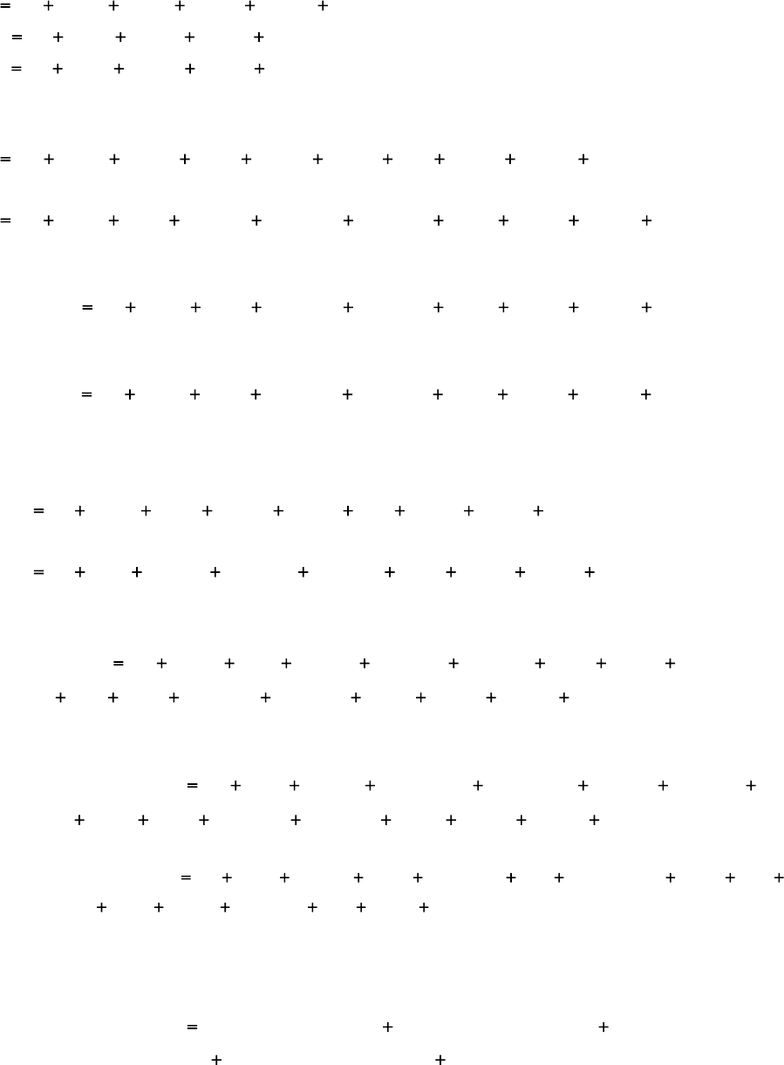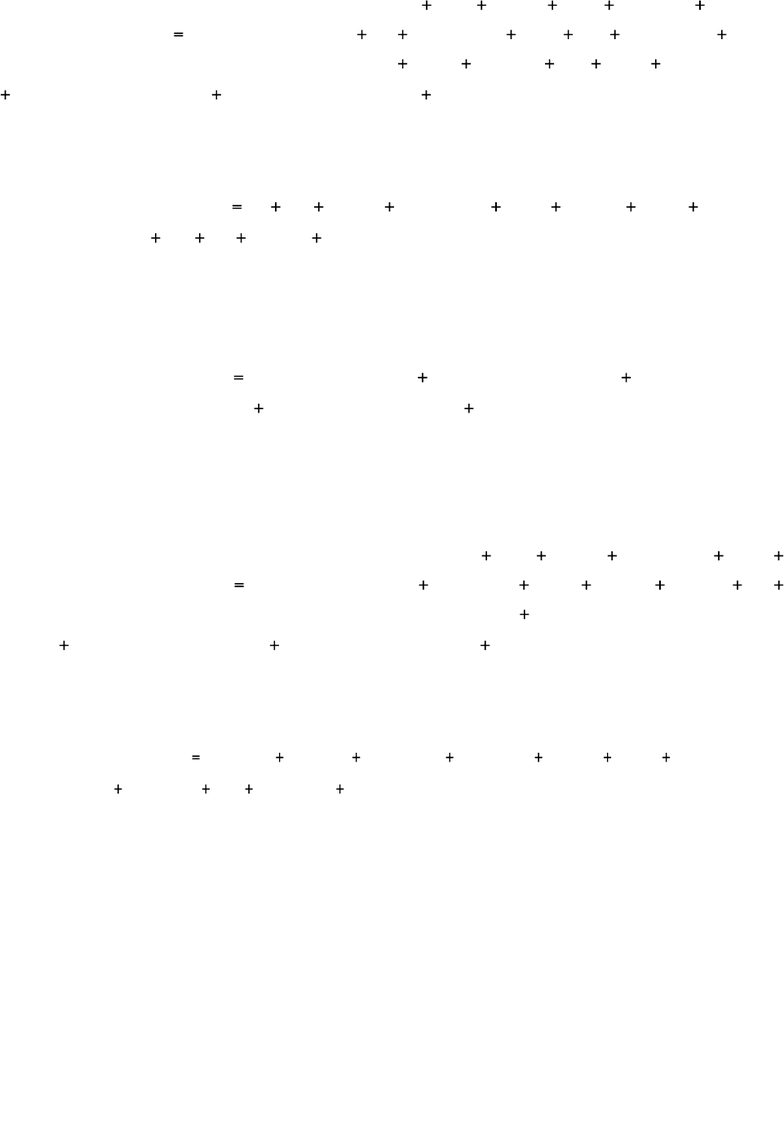Study Guides (390,000)
US (220,000)
KSU (400)
BUS (10)
Final

# BUS 10123 Chapter Notes - Chapter 7: Variance-Based Sensitivity Analysis, Simultaneous Equations, Jerry A. HausmanExam

Department
Course Code
BUS 10123
Professor
Eric Von Hendrix
Study Guide
Final

This preview shows pages 1-3. to view the full 9 pages of the document.1. (a) This is simple to accomplish in theory, but difficult in practice as a result
of the algebra. The original equations are (renumbering them (1), (2) and (3)
for simplicity)
( )
( )
( )
1
2
3
The easiest place to start (I think) is to take equation (1), and substitute in for y3t,
to get
Working out the products that arise when removing the brackets,
Gathering terms in y1t on the LHS:
(4)
Now substitute into (2) for y3t from (3).
Removing the brackets
(5)
Substituting into (4) for y2t from (5),
Taking the y1t terms to the LHS:
Gathering like-terms in the other variables together:
(6)
Multiplying all through equation (3) by :

Only pages 1-3 are available for preview. Some parts have been intentionally blurred.2
(7)
Replacing in (7) with the RHS of (6),
(8)
Expanding the brackets in equation (8) and cancelling the relevant terms
(9)
Multiplying all through equation (2) by :
(10)
Replacing in (10) with the RHS of (9),
(11)
Expanding the brackets in (11) and cancelling the relevant terms
(12)
Although it might not look like it (!), equations (6), (12), and (9) respectively
will give the reduced form equations corresponding to (1), (2), and (3), by
doing the necessary division to make y1t, y2t, or y3t the subject of the formula.
From (6),

Only pages 1-3 are available for preview. Some parts have been intentionally blurred.3
(13)
From (12),
(14)
From (9),
(15)
Notice that all of the reduced form equations (13)-(15) in this case depend on
all of the exogenous variables, which is not always the case, and that the
equations contain only exogenous variables on the RHS, which must be the
case for these to be reduced forms.
(b) The term “identificationrefers to whether or not it is in fact possible to
obtain the structural form coefficients (the
,
, and
’s in equations (1)-(3))
from the reduced form coefficients (the
’s) by substitution. An equation can
be over-identified, just-identified, or under-identified, and the equations in a
system can have differing orders of identification. If an equation is under-
identified (or not identified), then we cannot obtain the structural form
coefficients from the reduced forms using any technique. If it is just
identified, we can obtain unique structural form estimates by back-
substitution, while if it is over-identified, we cannot obtain unique structural
form estimates by substituting from the reduced forms.
There are two rules for determining the degree of identification of an
equation: the rank condition, and the order condition. The rank condition is a
necessary and sufficient condition for identification, so if the rule is satisfied,
it guarantees that the equation is indeed identified. The rule centres around a